# Shannon-Fano Algorithm for Data Compression

• Difficulty Level : Medium
• Last Updated : 07 Dec, 2021

DATA COMPRESSION AND ITS TYPES
Data Compression, also known as source coding, is the process of encoding or converting data in such a way that it consumes less memory space. Data compression reduces the number of resources required to store and transmit data.
It can be done in two ways- lossless compression and lossy compression. Lossy compression reduces the size of data by removing unnecessary information, while there is no data loss in lossless compression.

WHAT IS SHANNON FANO CODING?
Shannon Fano Algorithm is an entropy encoding technique for lossless data compression of multimedia. Named after Claude Shannon and Robert Fano, it assigns a code to each symbol based on their probabilities of occurrence. It is a variable-length encoding scheme, that is, the codes assigned to the symbols will be of varying length.

HOW DOES IT WORK?
The steps of the algorithm are as follows:

1. Create a list of probabilities or frequency counts for the given set of symbols so that the relative frequency of occurrence of each symbol is known.
2. Sort the list of symbols in decreasing order of probability, the most probable ones to the left and least probable to the right.
3. Split the list into two parts, with the total probability of both the parts being as close to each other as possible.
4. Assign the value 0 to the left part and 1 to the right part.
5. Repeat steps 3 and 4 for each part, until all the symbols are split into individual subgroups.

The Shannon codes are considered accurate if the code of each symbol is unique.

EXAMPLE:
The given task is to construct Shannon codes for the given set of symbols using the Shannon-Fano lossless compression technique.

Step: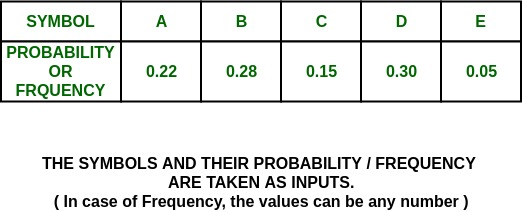Tree: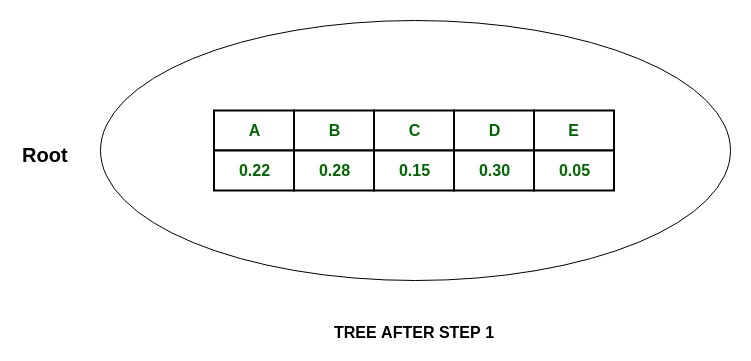Solution:

1. Upon arranging the symbols in decreasing order of probability:

P(D) + P(B) = 0.30 + 0.2 = 0.58

and,

P(A) + P(C) + P(E) = 0.22 + 0.15 + 0.05 = 0.42

And since the almost equally split the table, the most is divided it the blockquote table isblockquotento

{D, B} and {A, C, E}

and assign them the values 0 and 1 respectively.

Step: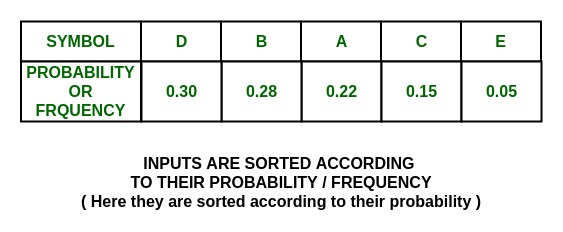Tree: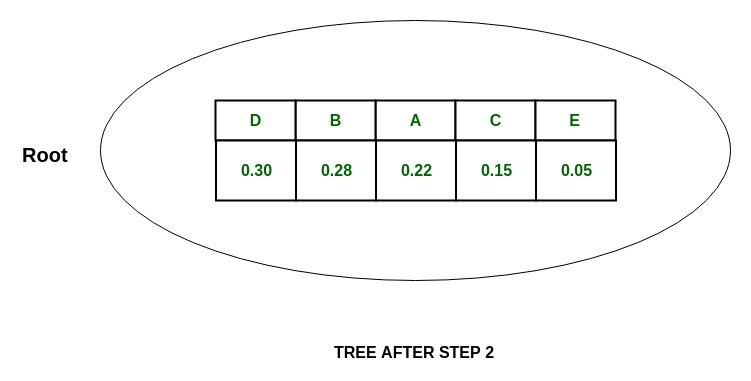2. Now, in {D, B} group,

P(D) = 0.30 and P(B) = 0.28

which means that P(D)~P(B), so divide {D, B} into {D} and {B} and assign 0 to D and 1 to B.

Step: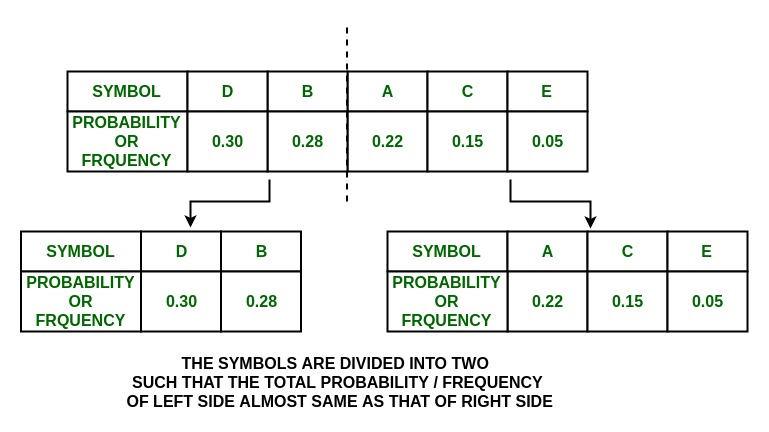Tree: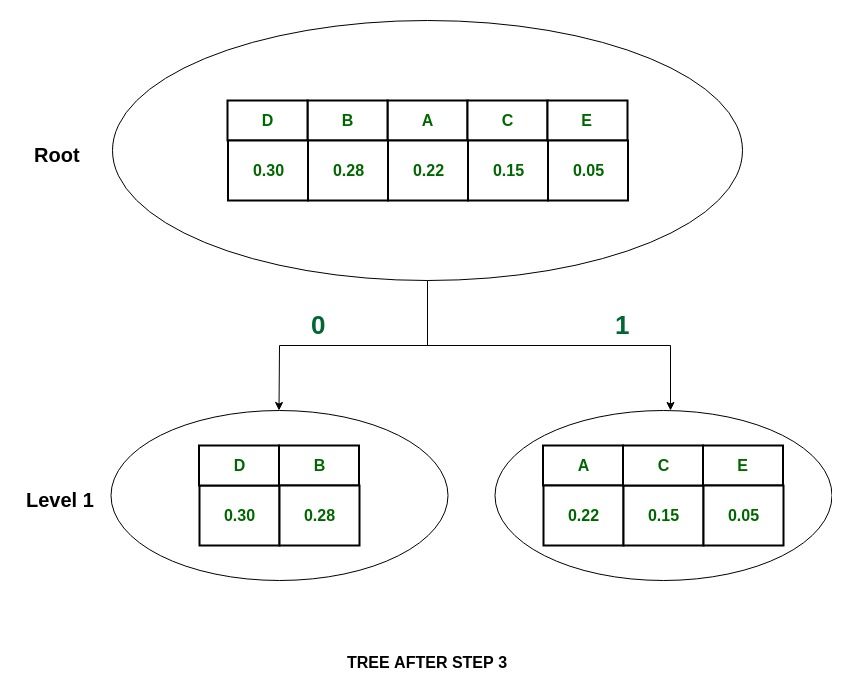3. In {A, C, E} group,

P(A) = 0.22 and P(C) + P(E) = 0.20

So the group is divided into

{A} and {C, E}

and they are assigned values 0 and 1 respectively.

4. In {C, E} group,

P(C) = 0.15 and P(E) = 0.05

So divide them into {C} and {E} and assign 0 to {C} and 1 to {E}

Step: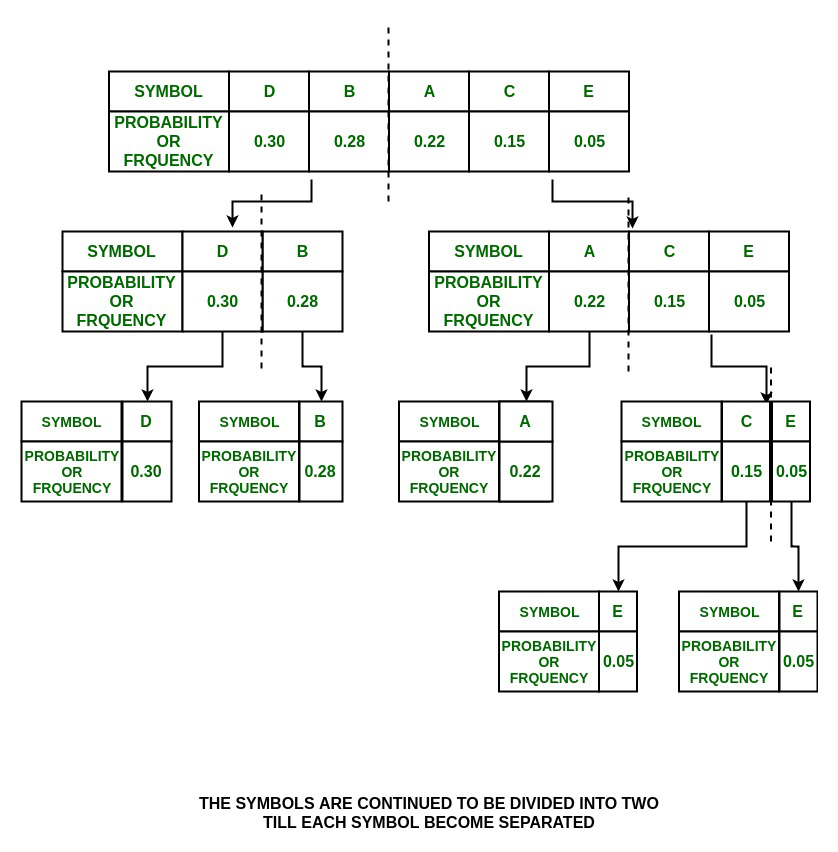Tree: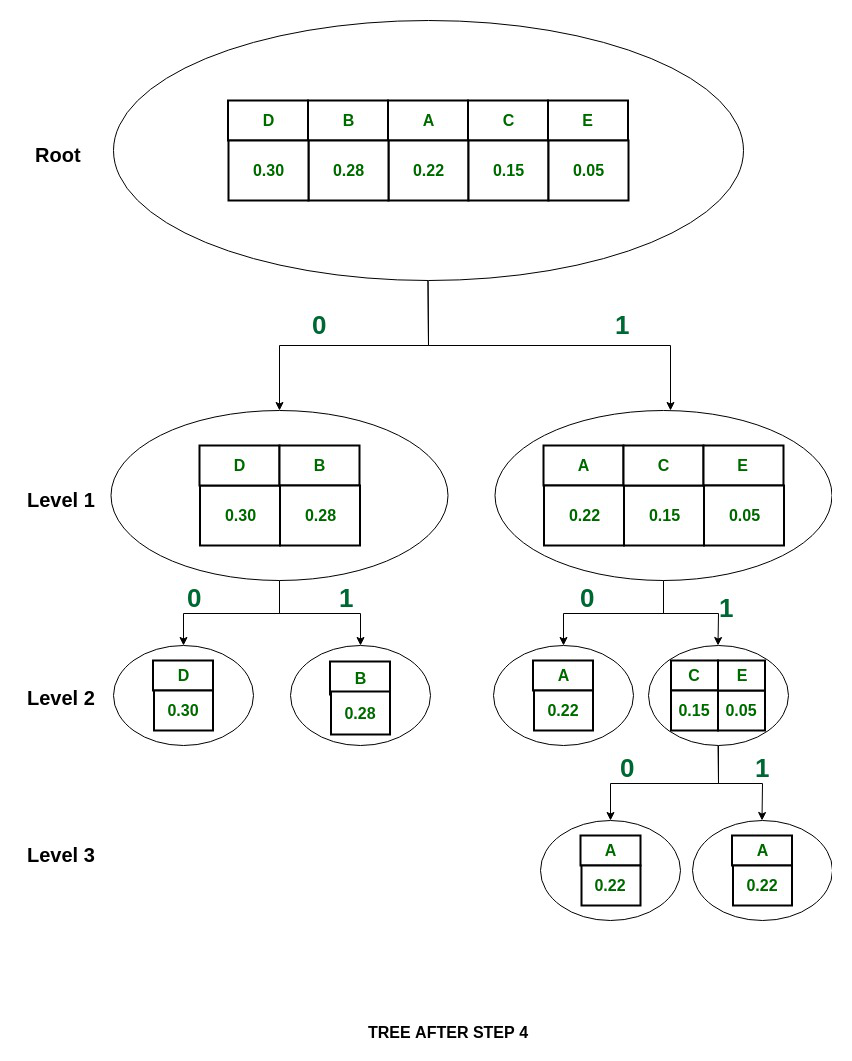Note: The splitting is now stopped as each symbol is separated now.

The Shannon codes for the set of symbols are: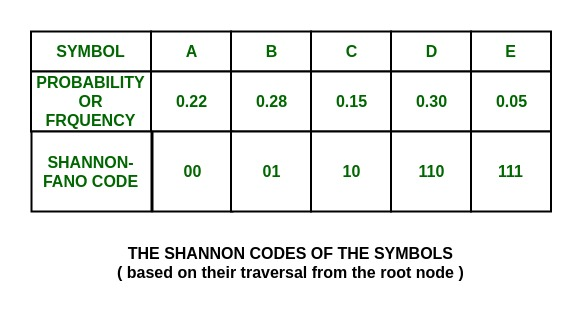As it can be seen, these are all unique and of varying lengths.

Below is the implementation of the above approach:

## C++

 `// C++ program for Shannon Fano Algorithm` `// include header files``#include ``using` `namespace` `std;` `// declare structure node``struct` `node {` `    ``// for storing symbol``    ``string sym;` `    ``// for storing probability or frequency``    ``float` `pro;``    ``int` `arr;``    ``int` `top;``} p;` `typedef` `struct` `node node;` `// function to find shannon code``void` `shannon(``int` `l, ``int` `h, node p[])``{``    ``float` `pack1 = 0, pack2 = 0, diff1 = 0, diff2 = 0;``    ``int` `i, d, k, j;``    ``if` `((l + 1) == h || l == h || l > h) {``        ``if` `(l == h || l > h)``            ``return``;``        ``p[h].arr[++(p[h].top)] = 0;``        ``p[l].arr[++(p[l].top)] = 1;``        ``return``;``    ``}``    ``else` `{``        ``for` `(i = l; i <= h - 1; i++)``            ``pack1 = pack1 + p[i].pro;``        ``pack2 = pack2 + p[h].pro;``        ``diff1 = pack1 - pack2;``        ``if` `(diff1 < 0)``            ``diff1 = diff1 * -1;``        ``j = 2;``        ``while` `(j != h - l + 1) {``            ``k = h - j;``            ``pack1 = pack2 = 0;``            ``for` `(i = l; i <= k; i++)``                ``pack1 = pack1 + p[i].pro;``            ``for` `(i = h; i > k; i--)``                ``pack2 = pack2 + p[i].pro;``            ``diff2 = pack1 - pack2;``            ``if` `(diff2 < 0)``                ``diff2 = diff2 * -1;``            ``if` `(diff2 >= diff1)``                ``break``;``            ``diff1 = diff2;``            ``j++;``        ``}``        ``k++;``        ``for` `(i = l; i <= k; i++)``            ``p[i].arr[++(p[i].top)] = 1;``        ``for` `(i = k + 1; i <= h; i++)``            ``p[i].arr[++(p[i].top)] = 0;` `        ``// Invoke shannon function``        ``shannon(l, k, p);``        ``shannon(k + 1, h, p);``    ``}``}` `// Function to sort the symbols``// based on their probability or frequency``void` `sortByProbability(``int` `n, node p[])``{``    ``int` `i, j;``    ``node temp;``    ``for` `(j = 1; j <= n - 1; j++) {``        ``for` `(i = 0; i < n - 1; i++) {``            ``if` `((p[i].pro) > (p[i + 1].pro)) {``                ``temp.pro = p[i].pro;``                ``temp.sym = p[i].sym;` `                ``p[i].pro = p[i + 1].pro;``                ``p[i].sym = p[i + 1].sym;` `                ``p[i + 1].pro = temp.pro;``                ``p[i + 1].sym = temp.sym;``            ``}``        ``}``    ``}``}` `// function to display shannon codes``void` `display(``int` `n, node p[])``{``    ``int` `i, j;``    ``cout << ``"\n\n\n\tSymbol\tProbability\tCode"``;``    ``for` `(i = n - 1; i >= 0; i--) {``        ``cout << ``"\n\t"` `<< p[i].sym << ``"\t\t"` `<< p[i].pro << ``"\t"``;``        ``for` `(j = 0; j <= p[i].top; j++)``            ``cout << p[i].arr[j];``    ``}``}` `// Driver code``int` `main()``{``    ``int` `n, i, j;``    ``float` `total = 0;``    ``string ch;``    ``node temp;` `    ``// Input number of symbols``    ``cout << ``"Enter number of symbols\t: "``;``    ``n = 5;``    ``cout << n << endl;` `    ``// Input symbols``    ``for` `(i = 0; i < n; i++) {``        ``cout << ``"Enter symbol "` `<< i + 1 << ``" : "``;``        ``ch = (``char``)(65 + i);``        ``cout << ch << endl;` `        ``// Insert the symbol to node``        ``p[i].sym += ch;``    ``}` `    ``// Input probability of symbols``    ``float` `x[] = { 0.22, 0.28, 0.15, 0.30, 0.05 };``    ``for` `(i = 0; i < n; i++) {``        ``cout << ``"\nEnter probability of "` `<< p[i].sym << ``" : "``;``        ``cout << x[i] << endl;` `        ``// Insert the value to node``        ``p[i].pro = x[i];``        ``total = total + p[i].pro;` `        ``// checking max probability``        ``if` `(total > 1) {``            ``cout << ``"Invalid. Enter new values"``;``            ``total = total - p[i].pro;``            ``i--;``        ``}``    ``}` `    ``p[i].pro = 1 - total;` `    ``// Sorting the symbols based on``    ``// their probability or frequency``    ``sortByProbability(n, p);` `    ``for` `(i = 0; i < n; i++)``        ``p[i].top = -1;` `    ``// Find the shannon code``    ``shannon(0, n - 1, p);` `    ``// Display the codes``    ``display(n, p);``    ``return` `0;``}`

## Python3

 `# Python3 program for Shannon Fano Algorithm`  `# declare structure node``class`  `node :``    ``def` `__init__(``self``) ``-``> ``None``:``        ``# for storing symbol``        ``self``.sym``=``''``        ``# for storing probability or frequency``        ``self``.pro``=``0.0``        ``self``.arr``=``[``0``]``*``20``        ``self``.top``=``0``p``=``[node() ``for` `_ ``in` `range``(``20``)]` `# function to find shannon code``def` `shannon(l, h, p):``    ``pack1 ``=` `0``; pack2 ``=` `0``; diff1 ``=` `0``; diff2 ``=` `0``    ``if` `((l ``+` `1``) ``=``=` `h ``or` `l ``=``=` `h ``or` `l > h) :``        ``if` `(l ``=``=` `h ``or` `l > h):``            ``return``        ``p[h].top``+``=``1``        ``p[h].arr[(p[h].top)] ``=` `0``        ``p[l].top``+``=``1``        ``p[l].arr[(p[l].top)] ``=` `1``        ` `        ``return``    ` `    ``else` `:``        ``for` `i ``in` `range``(l,h):``            ``pack1 ``=` `pack1 ``+` `p[i].pro``        ``pack2 ``=` `pack2 ``+` `p[h].pro``        ``diff1 ``=` `pack1 ``-` `pack2``        ``if` `(diff1 < ``0``):``            ``diff1 ``=` `diff1 ``*` `-``1``        ``j ``=` `2``        ``while` `(j !``=` `h ``-` `l ``+` `1``) :``            ``k ``=` `h ``-` `j``            ``pack1 ``=` `pack2 ``=` `0``            ``for` `i ``in` `range``(l, k``+``1``):``                ``pack1 ``=` `pack1 ``+` `p[i].pro``            ``for` `i ``in` `range``(h,k,``-``1``):``                ``pack2 ``=` `pack2 ``+` `p[i].pro``            ``diff2 ``=` `pack1 ``-` `pack2``            ``if` `(diff2 < ``0``):``                ``diff2 ``=` `diff2 ``*` `-``1``            ``if` `(diff2 >``=` `diff1):``                ``break``            ``diff1 ``=` `diff2``            ``j``+``=``1``        ` `        ``k``+``=``1``        ``for` `i ``in` `range``(l,k``+``1``):``            ``p[i].top``+``=``1``            ``p[i].arr[(p[i].top)] ``=` `1``            ` `        ``for` `i ``in` `range``(k ``+` `1``,h``+``1``):``            ``p[i].top``+``=``1``            ``p[i].arr[(p[i].top)] ``=` `0``            `  `        ``# Invoke shannon function``        ``shannon(l, k, p)``        ``shannon(k ``+` `1``, h, p)``    `   `# Function to sort the symbols``# based on their probability or frequency``def` `sortByProbability(n, p):``    ``temp``=``node()``    ``for` `j ``in` `range``(``1``,n) :``        ``for` `i ``in` `range``(n ``-` `1``) :``            ``if` `((p[i].pro) > (p[i ``+` `1``].pro)) :``                ``temp.pro ``=` `p[i].pro``                ``temp.sym ``=` `p[i].sym` `                ``p[i].pro ``=` `p[i ``+` `1``].pro``                ``p[i].sym ``=` `p[i ``+` `1``].sym` `                ``p[i ``+` `1``].pro ``=` `temp.pro``                ``p[i ``+` `1``].sym ``=` `temp.sym``            ` `        ` `    `   `# function to display shannon codes``def` `display(n, p):``    ``print``(``"\n\n\n\tSymbol\tProbability\tCode"``,end``=``'')``    ``for` `i ``in` `range``(n ``-` `1``,``-``1``,``-``1``):``        ``print``(``"\n\t"``, p[i].sym, ``"\t\t"``, p[i].pro,``"\t"``,end``=``'')``        ``for` `j ``in` `range``(p[i].top``+``1``):``            ``print``(p[i].arr[j],end``=``'')``    `   `# Driver code``if` `__name__ ``=``=` `'__main__'``:``    ``total ``=` `0` `    ``# Input number of symbols``    ``print``(``"Enter number of symbols\t: "``,end``=``'')``    ``n ``=` `5``    ``print``(n)``    ``i``=``0``    ``# Input symbols``    ``for` `i ``in` `range``(n):``        ``print``(``"Enter symbol"``, i ``+` `1``,``" : "``,end``=``"")``        ``ch ``=` `chr``(``65` `+` `i)``        ``print``(ch)` `        ``# Insert the symbol to node``        ``p[i].sym ``+``=` `ch``    `  `    ``# Input probability of symbols``    ``x ``=` `[``0.22``, ``0.28``, ``0.15``, ``0.30``, ``0.05``]``    ``for` `i ``in` `range``(n):``        ``print``(``"\nEnter probability of"``, p[i].sym, ``": "``,end``=``"")``        ``print``(x[i])` `        ``# Insert the value to node``        ``p[i].pro ``=` `x[i]``        ``total ``=` `total ``+` `p[i].pro` `        ``# checking max probability``        ``if` `(total > ``1``) :``            ``print``(``"Invalid. Enter new values"``)``            ``total ``=` `total ``-` `p[i].pro``            ``i``-``=``1``        ` `    ` `    ``i``+``=``1``    ``p[i].pro ``=` `1` `-` `total``    ``# Sorting the symbols based on``    ``# their probability or frequency``    ``sortByProbability(n, p)` `    ``for` `i ``in` `range``(n):``        ``p[i].top ``=` `-``1` `    ``# Find the shannon code``    ``shannon(``0``, n ``-` `1``, p)` `    ``# Display the codes``    ``display(n, p)`
Output:
```Enter number of symbols    : 5
Enter symbol 1 : A
Enter symbol 2 : B
Enter symbol 3 : C
Enter symbol 4 : D
Enter symbol 5 : E

Enter probability of A : 0.22

Enter probability of B : 0.28

Enter probability of C : 0.15

Enter probability of D : 0.3

Enter probability of E : 0.05

Symbol    Probability    Code
D        0.3    00
B        0.28    01
A        0.22    10
C        0.15    110
E        0.05    111```

My Personal Notes arrow_drop_up